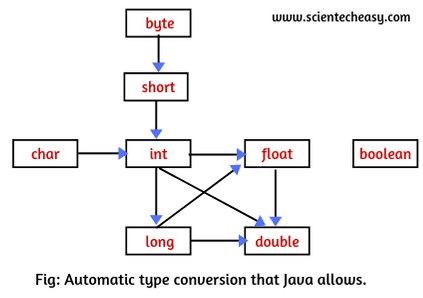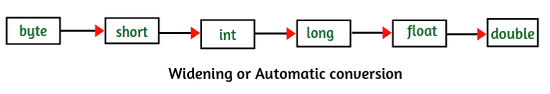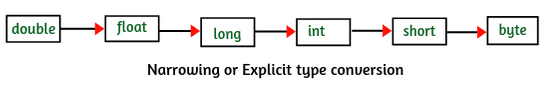# Type Conversion, Type Casting in Java with Example

## What is Type conversion and Type casting in Java?

The process of converting a value from one data type to another is known as type conversion. If the two data types are compatible with each other, Java will perform such conversion automatically or implicitly for you.

Automatic conversion done by java compiler internally is called implicit conversion or implicit casting. It is performed to convert a lower data type into a higher data type. Implicit conversion is also known as automatic type promotion in java.

For example, If you assign an int value to a long variable, It is compatible with each other but an int value cannot be assigned to a byte variable.

int x = 20; long y = x; // Automatic conversion byte z = x; // Type mismatch: cannot convert from int to byte.

The conversion of an int value to byte is an incompatible type and it cannot be performed automatically by Java compiler because a byte is smaller than an int.

Similarly, no automatic conversion will take place from double to byte. These kinds of conversion can be done by using a technique called type casting in java

Type casting performs an explicit conversion between incompatible types. Therefore, it is also known as an explicit type casting in Java. It is used to an object or variable of one type to another. It must be done explicitly by the programmer. The compiler will not do it automatically. Let’s understand in more detail both automatic type conversions and type casting.

## Automatic Type Conversion in Java with Example

When you perform arithmetic or mathematical operations with operands of different types, Java compiler performs an implicit conversion internally i.e it automatically converts the ‘lower’ type to the ‘higher’ type before the operation proceeds and gives the result of higher type.

Lower types are those types which consume less memory and store less number of digits in a value. Higher types use more memory and store more number of digits.

The below figure shows which conversion is allowed by Java. Let’s understand the concept from the diagram.From the above diagram, a byte can be promoted to short, int, long, float or double. Similarly, the short data type can be promoted to int, long, float or double. The char data type can be promoted to int, float, long or double and so on.

### Automatic Type Promotion Rules in Expression

1. If byte, short, and int are used in a mathematical expression, Java always converts the result into an int.

2. If a single long is used in the expression, the whole expression is converted to long.
3. If a float operand is used in an expression, the whole expression is converted to float.
4. If any operand is double, the result is promoted to double.

5. Boolean values cannot be converted to another type.
6. Conversion from float to int causes truncation of the fractional part which represents the loss of precision. Java does not allow this.

7. Conversion from double to float causes rounding of digits that may cause some of the value’s precision to be lost.
8. Conversion from long to int is also not possible. It causes dropping of the excess higher order bits.

For example, when you will perform the addition of an int and a double, it gives the result in a double and the subtraction of a long from a float results in a float.

Let’s understand the concept in the below program.
Program source code 1:

```package typeConversion;
public class AutoTypeConversion
{
int x = 20;
double y = 40.5;
long p = 30;
float q = 10.60f;
void sum()
{
// int z = x + y; (1) // Error; cannot convert from double to int.
double z = x + y;

System.out.println("Sum of two numbers: " +z);
}
void sub()
{
// long r = p - q; // (2) // Error; cannot convert from float to long.
float r = p - q;
System.out.println("Subtraction of two numbers: " +r);
}
public static void main(String[] args)
{
AutoTypeConversion obj = new AutoTypeConversion();
obj.sum(); // Calling sum method.
obj.sub(); // Calling sub method.
}
}```
```Output:
Sum of two numbers: 60.5
Subtraction of two numbers: 19.4```

Explanation: In the above example, the result of the addition is double because when an int and a double are added, the int is promoted to the higher-ranking data type double. Therefore, assigning the result as a double is legal.

But when the result will assign to be an int, the fractional part of the value of y will be truncated and the result will give the value of 60, not 60.5. It represents a loss of precision and Java does not allow any loss of precision. Therefore, the compiler will generate an error in line number 1.

Similarly, the result of the subtraction is float because when long and float are subtracted, the long is promoted to the higher-ranking data type float.

Let’s take one more example program to understand automatic type promotion concept in java.
Program source code 2:

```package typeConversion;
public class PromoteTest
{
public static void main(String[] args)
{
byte b = 42;
char c = 'a';
short s = 1024;
int i = 50000;
float f = 5.67f;
double d = .1234;

// Expression:
double result = (f * b) + (i / c) - (d * s);

//Result after all the promotions are done.
System.out.println("result = " + result);
}
}```
```Output:
result = 626.7784146484375```

Explanation:
1. In the first sub expression, f * b, b is promoted to float and the result of the sub expression is float.
2. In the second sub expression i / c, first c is promoted to int and the result of the sub expression will be int.
3. Then, in d * s, the value of s is promoted to double and the data type of sub expression is double.

Finally, now we will consider these three intermediate values with data type float, int, and double. When the addition of a float and an int is performed, the outcome is float. Then, the resultant float is minus with the last double, is converted to double, which is the data type of the final result of the expression.

### When takes place Automatic type conversion in Java?

An automatic type conversion takes place in Java if the following two conditions are met:
1. The data types are compatible with each other.
2. The destination type is bigger than the source type.

When these two conditions are met, a widening conversion will take place. The process of converting lower data type into higher data type is called widening. For example, the int type is always large enough to hold all bytes value. Therefore, no explicit casting is needed.

The widening conversion (i.e automatic type conversion) is possible in Java when the numeric type (including integer and floating point types) are compatible with each other but no widening conversion is supported from numeric type to char or boolean because the numeric type is incompatible with char or boolean. Also char and boolean are incompatible with each other.

The following diagram is useful to understand the concept of widening conversion.Let’s understand the concept of widening conversion by an example program.
Program source code 2:

```package typeConversion;
public class WideningConversionEx
{
public static void main(String[] args)
{
int a = 50;
double d = 100;
// Automatic type conversion
long l = a;
// int i = l; // Type mismatch: cannot convert from long to int.

// Automatic type conversion
float f = l;
// int i1 = f; // Type mismatch: cannot convert from float to int.
// float f1 = d; // Type mismatch.
double d1 = f;

System.out.println("Int value "+a);
System.out.println("Long value "+l);
System.out.println("Float value "+f);
System.out.println("Double value " +d1);
}
}```
```Output:
Int value 50
Long value 50
Float value 50.0
Double value 50.0```

In the preceding program, the long type is large enough to hold int value but an int type cannot enough to hold long type because int is smaller than long. Similarly, float type is large enough to hold long type but int type cannot hold a float value.

As you will observe in the above program, there is no any loss of data or precision or accuracy, therefore, widening conversion is safe. This is reason, java compiler does widening conversion automatically. This conversion is also called implicit casting in java.

## Explicit type casting (Narrowing conversion) in Java

As we know that automatic conversion is very helpful and safe, but they cannot fulfill all the needs. For example, if you will assign a double value to an int variable, this conversion cannot be performed automatically because an int is smaller than a double.

In this case, you must use casting to create a conversion between two incompatible types. This kind of conversion is also known as narrowing conversion. The conversion of a higher data type into a lower data type is called narrowing conversion.

Since this type of conversion is performed by the programmer, not by the compiler automatically, therefore, it is also called explicit type casting in java. It is done to convert from higher data type to a lower data type.

The following diagram is useful to perform the narrowing conversion or explicit type casting.To perform this kind of type casting, you will have to use a cast operator. A cast operator is used to cast a primitive value from one type to another. Using a cast operator is simply an explicit type conversion. The general form of a cast is given below:
Syntax:
(type_name) expression;
Where type_name specify a standard data type. The expression may be a variable, constant or an expression.

Let’s take different types of conversion to understand the concept of explicit type casting in java.
1. int x;
double y = 9.99;
x = (int)y;   // Now, it will compile in Java and the resulting value will simply be 9.

Here, we are converting double type into int type by using cast operator (int) before variable y. The value in y is 9.99. Since it is converting into int data type, the fractional part of a double will be discarded when casting to an integer. It is not rounded.

Only 9 will be stored in x. Now, observe that we are losing some digits. So, narrowing conversion is not safe. That’s why Java compiler forces programmer to use cast operator when going for narrowing or explicit casting.

2. double d = 100.9;
long l = (long)d; // Explicit type casting. The output will be 100 because the fractional part is lost.

3. int x;
float y = 27.6f;
x = (int)(y + 0.5); // On explicit type casting, the output becomes 28.

4. int a = 66;
char ch = (char)a; // ch stores ‘B’.
In this example, we are converting int type a value into char type. When the value of n is converted into char type, ch will store a character ‘B’ because value 66 of n is the ASCII value of ‘B’.

Let’s take example programs based on explicit type casting or narrowing conversion.
Program source code 3:

```package typeConversion;
public class ExplicitTest
{
double d = 100.04;
void conversion()
{
// explicit type casting
long l = (long)d;
int i = (int)l;
System.out.println("Double value "+d); // fractional part lost.
System.out.println("Long value "+l); // fractional part lost.
System.out.println("Int value "+i);
}
public static void main(String[] args)
{
ExplicitTest obj = new ExplicitTest();
obj.conversion();
}
}```
```Output:
Double value 100.04
Long value 100
Int value 100```

Program source code 4:

```package typeConversion;
public class ExplicitTest
{
public static void main(String[] args)
{
byte b;
int i = 257;
double d = 323.142;
System.out.println("Conversion of int to byte.");
b = (byte) i;
System.out.println("i = " + i + " b = " + b);
System.out.println("nConversion of double to int.");

i = (int) d;
System.out.println("d = " + d + " b = " + i);
System.out.println("nConversion of double to byte.");

b = (byte) d;
System.out.println("d = " + d + " b = " + b);
}
}
```
```Output:
Conversion of int to byte.
i = 257
b = 1
Conversion of double to int.
d = 323.142
b = 323
Conversion of double to byte.
d = 323.142
b = 67```

In this example program, when the value 257 is cast into a byte variable, the output is 1 that is the remainder of the division of 257 by 256 (the range of a byte). When d is converted into an int, its fractional part is lost. When d is converted into a byte, its fractional part is lost and the value is reduced module 256, that is 67 in this case.

## Disadvantage of Explicit Type casting in Java

There are the following disadvantages of using type casting in Java.
1. When you will use type casting in Java, you can lose some information or data.
2. Accuracy can be lost while using type casting.
3. When a double is cast to an int, the fractional part of a double is discarded which causes the loss of fractional part of data.

## Difference between Type conversion and Type casting in Java

The differences between type conversion and type casting in java are as follows:
1. Type conversion is also called implicit casting whereas, type casting is also called explicit casting.
2. Automatic type conversion is done internally by java compiler whereas, explicit type casting is done by the programmer. Compiler does not perform it automatically.
3. In casting, cast operator is needed whereas, no need for any operator needs in case of type conversion.
4. If you perform type casting in a program, you can lose information or data but in case of automatic type conversion, there is no loss of data.
5. Accuracy is not maintained in type casting whereas, there is no issue of accuracy in type conversion.
6. Type conversion is safe but type casting is not safe.

Final words
Hope that this tutorial has covered all the important points related to type conversion and type casting in Java with example programs. I hope that you will have understood type casting in java and enjoyed programming.